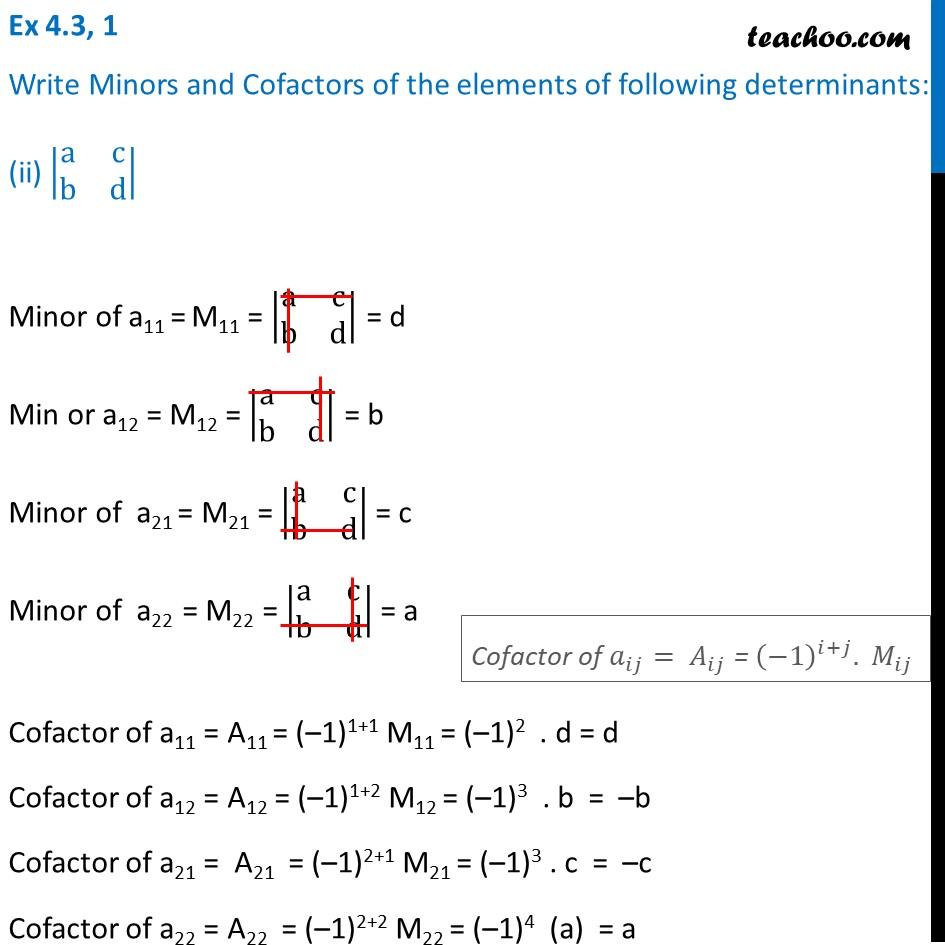Ex 4.3

Chapter 4 Class 12 Determinants
Serial order wiseLearn in your speed, with individual attention - Teachoo Maths 1-on-1 Class

### Transcript

Ex 4.3, 1 Write Minors and Cofactors of the elements of following determinants: (ii) |■8(a&c@b&d)| Minor of a11 = M11 = |■8(a&c@b&d)| = d Min or a12 = M12 = |■8(a&c@b&d)| = b Minor of a21 = M21 = |■8(a&c@b&d)| = c Minor of a22 = M22 = |■8(a&c@b&d)| = a Cofactor of a11 = A11 = (–1)1+1 M11 = (–1)2 . d = d Cofactor of a12 = A12 = (–1)1+2 M12 = (–1)3 . b = –b Cofactor of a21 = A21 = (–1)2+1 M21 = (–1)3 . c = –c Cofactor of a22 = A22 = (–1)2+2 M22 = (–1)4 (a) = a